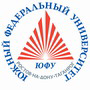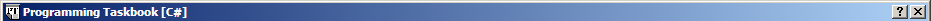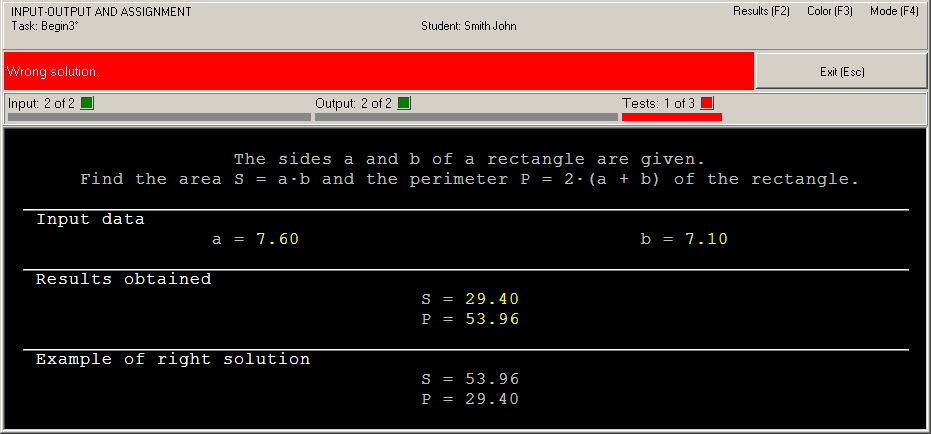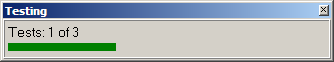Russian©  M. E. Abramyan (Southern Federal University, Shenzhen MSU-BIT University), 1998–2023

 Main Tasks Examples PT for MPI-2

 Overview PascalABC.NET Brief info PT4 types and procedures Simple task Pascal Brief info Configuring IDE PT4 types and procedures Simple task C and C++ Brief info Configuring IDE PT4 types and functions PT4 types and functions for C++ before version 4.23 Simple task C#, VB.NET, F# Brief info Configuring IDE PT4 methods Simple task Python Brief info Configuring IDE PT4 types and functions Simple task Java Brief info Configuring IDE PT4 classes Simple task Ruby and Julia Brief info Configuring IDE PT4 types and functions Simple task
 Examples | C#, VB.NET, F# | Simple task

# Solution of the simple task: Begin3

This section contains description of solving the following simple task in C#, VB.NET, and F#.

Begin3°. The sides a and b of a rectangle are given. Find the area S = ab and the perimeter P = 2(a + b) of the rectangle.

We shall use Visual Studio 2017 as programming environment, however the same results may be received in the Visual Studio 2019, 2022, SharpDevelop, and VS Code IDEs.

In this and the following sections we shall use the "procedure" notion not only for VB.NET subroutines but also for C# and F# functions with the void return type.

### Creating a template and acquaintance with the task

To create a template of the required task you should use the PT4Load tool (starting with version 4.22, you can use the PT4Panel tool for quick launch of all Programming Taskbook tools; the PT4Panel shortcut is located on the desktop and in any working directory).

The template created for Visual Studio 2017 consists of several files. But we need only Begin.cs file (for C#) or Begin3.vb file (for VB.NET) or Begin3.fs file (for F#). This file will be loaded into the editor of Visual Studio:

[Begin3.cs]

```// File: "Begin3"
using PT4;
using System;
using System.Collections.Generic;
using System.Linq;
using System.Text;

{
{
public static void Solve()
{

}
}
}
```

[Begin3.vb]

```Option Strict On
Option Infer On
Imports PT4.PTVB
Imports System
Imports System.Collections.Generic
Imports System.Linq
Imports System.Text

Sub Solve()

End Sub
End Module
```

[Begin3.fs]

```module MyTask
open PT4
open System
open System.Collections.Generic
open System.Linq
open System.Text

```

The Begin3.cs file contains definition of the MyTask class; this class is a descendant of the PT class defined in the PT4 namespace. The MyTask class includes the Solve procedure as its method. The Solve procedure already contains the Task procedure call that initializes the Begin3 task. The Begin3.vb file contains definition of the Solve procedure; this procedure already contains the Task procedure call. The solution of the task should be entered in the Solve procedure (of course any other procedures and functions may be used). The Begin3.fs file contains the description of the Solve variable, which includes a call to the Task procedure (when calling procedures and functions of the taskbook in F# programs, you must specify the name of the pt class in which they are defined before them). The solution to the problem should be placed after the line with the description of the Solve variable.

Note that the Task procedure (just as Get-functions and the Put procedure) is a static method of the PT class for C#, PTVB class for VB.NET, and pt class for F# (all these methods are declared with the "static" attribute in C# and the "Shared" attribute in VB.NET). In C#, all these methods are called in the Solve method of the MyTask class (that is a descendant of the PT class), therefore it is not necessary to qualify these methods with the PT prefix. In VB.NET the PTVB prefix is not necessary due to the "Imports PT4.PTVB" directive.

Also note that definition of Main procedure is absent in the Begin3.cs and Begin3.vb files. The Main procedure is defined in the pt4main file that was created when the project was generated for this task (the pt4main file has .cs extension for C#, .vb extension for VB.NET, and .fs extension for F#). The pt4main file is not loaded into the editor of Visual Studio. But the project includes this file (see the Solution Explorer window located on the Visual Studio window).

The project also includes the pt4net.dll library. This library contains classes used by Programming Taskbook.

To run the program, press [F5]. When the program is launched you will see the Programming Task window with a task text and initial data. Here is the screenshot of the window for the C# language; the window for VB.NET and F# has the "[Visual Basic.NET]" and "[F#]" caption instead of "[C#]".This running is considered as acquaintance running because the program does not perform input-output operations. To close the Programming Taskbook window click the Exit button or press [Esc] or [F5] key.

### Initial data input

Before solving tasks you should input initial data in the program. In the Begin3 task the initial data are real numbers a and b that refer to sides of the rectangle.

The initial data should be input in the variable of the required type, otherwise Programming Taskbook will detect error. Let's model this situation in our program. For this purpose we shall organize initial data input to variables of the integer type using the GetInt function:

[C#]

```public static void Solve()
{
int a = GetInt(), b = GetInt();
}
```

[VB.NET]

```Sub Solve()
Dim a = GetInt(), b = GetInt()
End Sub
```

[F#]

```let Solve = pt.Task "Begin3"
let a = pt.GetInt()
let b = pt.GetInt()
```

Run the program once again. You will see the new set of initial data. For each running of the program a new initial data are generated, so it is necessary to develop an algorithm that processes correctly all admissible sets of initial data.In this case the running leads to the following information in the status bar: "Invalid type is used for an input data item." Note that each type of errors is marked by different color.

To input data correctly we should use the GetDouble function that provides input data of double type. Change our program as follows:

[C#]

```public static void Solve()
{
double a = GetDouble(), b = GetDouble();
}
```

[VB.NET]

```Sub Solve()
Dim a = GetDouble(), b = GetDouble()
End Sub
```

[F#]

```let Solve = pt.Task "Begin3"
let a = pt.GetDouble()
let b = pt.GetDouble()
```

Now data input is performed correctly, but the program does not output results. In such situation we have the following message: "Correct data input: all required data are input, no data are output" (Programming Taskbook version 4.15 or higher) or "Some data are not output" (previous versions).

### Calculation and output results

Let's perform the required calculations and output results using the Put procedure:

[C#]

```public static void Solve()
{
double a = GetDouble(), b = GetDouble(),
S = a * b, P = 2 * (a + b);
Put(P, S);
}
```

[VB.NET]

```Sub Solve()
Dim a = GetDouble(), b = GetDouble(), _
S = a * b, P = 2 * (a + b)
Put(P, S)
End Sub
```

[F#]

```let Solve = pt.Task "Begin3"
let a = pt.GetDouble()
let b = pt.GetDouble()
let S = a * b
let P = 2. * (a + b)
pt.Put(P, S)
```

When the program is running you can see output values at the panel of results. Because we output data in inverse order, the status bar contains the error message "Wrong solution".### Correct solution and testing

To correct the last error it is enough to change order of arguments in the Put procedure call:

[C#]

```    Put(S, P);
```

[VB.NET]

```    Put(S, P)
```

[F#]

```pt.Put(S, P)
```

When this program is running you will see the testing panel on screen:This panel appears when the program processes successfully at least one set of input data. The progress bar shows the amount of tests which are already performed, the text above the bar allows to determine how much tests should be performed successfully. The program testing finishes in two cases: when all required tests are performed successfully or when some test is failed. If the program is in infinite loop then you should close the testing panel by means of the [x] button in its upper right corner.

In our case the algorithm is correct, therefore the message "The task is solved!" will be shown.To browse information about the task solving you can use PT4Results tool (you may run this tool by pressing the [F2] key or clicking the Results label in the upper-right corner of the Programming Taskbook window):

```Begin3      S23/02 20:13 Acquaintance with the task.
Begin3      S04/02 15:07 Invalid type is used for an input data item.
Begin3      S04/02 15:09 Correct data input.
Begin3      S04/02 15:12 Wrong solution.
Begin3      S04/02 15:15 The task is solved!
```

The letter "S" denotes the programming language being used (C Sharp).

Remark. It is not necessary to use additional variables S and P, because you can output expressions:

[C#]

```public static void Solve()
{
double a = GetDouble(), b = GetDouble();
Put(a * b, 2 * (a + b));
}
```

[VB.NET]

```Sub Solve()
Dim a = GetDouble(), b = GetDouble()
Put(a * b, 2 * (a + b))
End Sub
```

[F#]

```let Solve = pt.Task "Begin3"
let a = pt.GetDouble()
let b = pt.GetDouble()
pt.Put(a * b, 2. * (a + b))
```

In addition, you can use the GetDouble2 function in the solution, which allows you to immediately input two initial real numbers:

[C#]

```public static void Solve()
{
var (a, b) = GetDouble2();
Put(a * b, 2 * (a + b));
}
```

[VB.NET]

```Sub Solve()
Dim ab = GetDouble2()
Dim a = ab.Item1, b = ab.Item2
Put(a * b, 2 * (a + b))
End Sub
```

[F#]

```let Solve = pt.Task "Begin3"
let a, b = pt.GetDouble2()
pt.Put(a * b, 2. * (a + b))
```Designed byM. E. Abramyan and V. N. Braguilevsky Last revised:04.04.2023